## Abstract

Two-dimensional glass model experiments are used to investigate the residual trapping mechanism of CO2 stored in saline aquifers. For this purpose, two proxy fluids are chosen to simulate the CO2-brine behavior under reservoir conditions. The first set of experiments is carried out by flooding n-heptane in a mixture of glycerol and water inside a glass bead porous media. Fluids and porous materials are designed so that the dimensionless groups are in the range of real storage sites. Another set of proxy fluid consists of dodecane and a different mixture of glycerol and water, representing the second wettability condition for the system. The size of the glass beads chosen was fine (70–110 μm) in order to investigate residual trapping phenomena. For each set, after complete drainage process, an imbibition process is performed and in each time step, images are taken from the phenomena. The images are processed using a red, green, blue (RGB) color concept using a Matlab code that was developed for this study. By using this process, it is possible to measure the residual trapping of CO2 proxy fluid for each test and to determine the saturation profile in the model. Tests are carried out at various imbibition and drainage rates to study the effect of the rate on the results. Fine-scale numerical simulation models are constructed for comparison with experimental results. Good agreement is obtained between the simulation results and the image processing estimations, as well as the readings from the material balances during the experiments. This study could provide a framework for modeling different reservoir conditions for residual trapping mechanism and the impact of different parameters in future studies.

## Introduction

A proposed strategy for the reduction in atmospheric CO2 emissions is CO2 capture and storage [1, 2]. CO2 storage in geological formations is considered as an important solution for preventing CO2 emissions; however, it is essential that this conducted safely and securely [1, 2]. The success of storage projects is highly dependent on understanding the process at reservoir conditions. There are other potential geological storage possibilities including depleted oil and gas reservoirs and coal beds, but the main attraction of brine formations as suitable storage sites for CO2 is better availability and larger potential volumes .

After CO2 is injected into aquifer formations, it is trapped into the reservoir through various mechanisms, where each trapping mechanism generally has a different timescale. At the start of the injection period, a structural or geological mechanism dominates, where CO2 is trapped below a sealing cap rock . During the movement of CO2 upward or along a dipping barrier, brine will imbibe the formation that already has CO2 inside and some of the CO2 will be trapped in the pore spaces. This is called residual trapping. This study specifically investigates this phenomenon through visualization of the experiment and numerical simulation methods.

A successful CO2 storage project in an aquifer requires realistic modeling of CO2 behavior in the subsurface formation. The relative permeability and capillary pressure of the CO2-brine systems are important parameters in modeling residual trapping mechanisms. Several studies of relative permeability and capillary pressure in CO2-brine systems are reported [4-6]. Recent investigations of CO2-brine systems, including experimental capillary pressure and relative permeability studies, have been reported by the Benson research team at Stanford [4, 5].

Visualization methods are used extensively for recording results of different research studies. Some studies have applied 2D glass model experiments, especially for CO2 enhances oil recovery, heavy oil recovery techniques and cross-flow phenomena [7-9]. However, the application of 2D glass models for CO2 storage in brine is limited because of the unfavorable viscosity ratio and density difference that makes the process difficult to control and quantify. Lenormand et al.  studied stable displacement, viscous fingering, and capillary fingering in porous materials by use of micromodels. They have categorized the phenomena based on dimensionless parameters. In their work, although brine and CO2 are not studied, displacement experiments provide a good reference for the visualization methods . However, interpretation of the results in that work was done at microscopic level. Cinar et al. , performed 2ِD glass model flooding experiments to evaluate the effects of gravitational, viscous, and capillary forces in CO2 injection processes. The objective of that study was to investigate types of flow regimes that occur at different conditions of density and viscosity. The results of that study show the existence of pore-scale instabilities because of unfavorable viscosities and densities . Cinar et al.s studies were limited to drainage processes with emphasis on flow-type regimes. Later, Polak et al. (2011) , have reported 2D experimental investigations on the balance of forces during CO2 injection in the brine. They used 0.2 and 0.4 mm glass beads, and various injection rates were applied during the drainage process. The experiments are limited to drainage with capillary numbers in the range of 10−6. All the previous works focus on drainage processes.

In this study, 2D visual glass model experiments containing both drainage and imbibition processes within the range of CO2 storage in the reservoir are designed and carried out. A specific experimental design makes it possible to study the residual trapping in imbibition process. Selecting very fine glass beads and low-injection rates make it possible to perform the experiment in the range of capillary number of 10−7 and to investigate residual trapping phenomena. At these conditions flooding behavior is dominated by capillary forces. The idea is to quantify the saturations during the experiment using an image analysis method in both drainage and imbibition processes. Characterizing the saturation and flow patterns by use of an image analysis method is especially important where residual trapping is targeted. This allows the evaluation of the effect of different parameters such as the effect of injection rate and wettability. The impact of wettability is studied by the use of two sets of proxy fluids at different wettability conditions.

## Methods

### Image analysis

An image may be defined as a two-dimensional function f(x,y), where x and y are spatial coordinates and the value of f at any pair of coordinates is the intensity at that point . Digital technology has made it possible to manipulate multidimensional signals . The goal of this manipulation can be divided into three categories: Image processing (image in: image out), Image analysis (image in: measurements out), Image understanding (image in: high-level description out) . According to these definitions this study uses the fundamental image analysis and image understanding.

Color images are formed by a combination of individual images. For example in the RGB color definition, a color image consists of three individual mono chrome images, labeled as the red (R), green (G), and blue (B) primary colors . For this reason, many of the image analysis techniques are based on processing the three color components in the image. In this study, CO2 proxy fluid is dyed in red and the RGB color concept is used to develop a technique to estimate the fluid saturations from the images. The input is high quality images from the 2D model and the output is saturation measurements obtained from RGB color intensity technique. For every individual pixel the intensity of red, green, and blue colors are recorded, and by using a Matlab code and defining a function for absorption of the blue and green colors, it is possible to correlate it with the saturation. It is very important to define a base image in order to compare the images at each time step. Here, the red intensity shows the CO2 proxy saturation in the model. In the base image (before injection), the image of the porous material filled with brine proxy fluid can have an intensity of red color, however, the CO2 saturation function is defined relative to the base images. A specific Matlab code is developed for this purpose and each set of experiments is interpreted by use of the code.

### Scaling the experiment

Here, a one-dimensional, two-phase system for immiscible and incompressible flow of CO2 in a narrow porous channel filled with brine is considered. By introducing dimensionless parameters, it is possible to determine a set of dimensionless numbers for the problem. The medium is initially filled with the brine at SW = 1 and it is very long compared to the thickness of the reservoir, and has a dip angle of α. Two typical relative permeability curves with the end point relative permeability values of ${\textstyle k_{{rCO}_{2}}^{_{\ast }},k_{rw}^{_{\ast }}}$ and a capillary pressure curve with the maximum pressure of ${\textstyle P_{c}^{_{\ast }}}$ are considered for this problem . To develop the governing equation, conservation of mass and the Darcy equation have been normalized based on dimensionless length, time, porosity, and flux. By simplifying the equation and rearranging, three main dimensionless groups are derived . These three numbers are defining the physics of the problem.

 $N_{cr}={\frac {P_{c}^{_{\ast }}k_{rCO_{2}}^{_{\ast }}{\overline {k}}}{QL{\mu }_{CO_{2}}}}$
(1)
 $N_{g}={\frac {\Delta \rho gsin\alpha k_{rco_{2}}^{_{\ast }}{\overline {k}}}{Q{\mu }_{co_{2}}}}$
(2)
 $M={\frac {k_{rw/{\mu }_{w}}^{_{\ast }}}{k_{rco_{2}/{\mu }_{co_{2}}}^{_{\ast }}}}$
(3)

${\textstyle {\mu }_{CO_{2}}}$ and μw are viscosities of CO2 and brine, respectively. Q is injection flux (m/sec) and ${\textstyle {\overline {k}}}$ is average permeability (m2) and L is length of the model (m). Ncr is a dimensionless parameter that defines the effect of capillary to viscous forces. Ng defines the effect of gravity forces to viscous forces and M is mobility ratio. Gupta  has introduced the following capillary number for small-scale environments:

 $N_{c}={\frac {U\mu }{\gamma Cos(\theta )}}$
(4)

Here, γ is interfacial tension (IFT) between two fluids and U is characteristics velocity and θ is contact angle between two fluids and porous material. The effect of contact angle is not considered in the basic definition of the capillary number but in this study this modified number is used. The capillary-to-gravity ratio (CGR) has been also defined to provide quantification of the relative effect of gravity in the presence of capillary pressure. CGR definition is first given by Iffly et al.  as follows:

 $N_{gr}={\frac {2\gamma Cos(\theta )}{\Delta \rho gL{\sqrt {\frac {k}{\varphi }}}}}$
(5)

Here is porosity of the media, in this study we use equation 3, 4, and 5 to be able to screen the available CO2 storage conditions and compare them with the experiment design. Equations 6 and 7 show CGR and capillary numbers without considering wettability effect:

 $N_{c}={\frac {U\mu }{\gamma }}$
(6)
 $N_{gr}={\frac {2\gamma }{\Delta \rho gL{\sqrt {\frac {k}{\varphi }}}}}$
(7)

### Fine grid reservoir simulation

Fine grid simulations (3.1*8*3.1 mm grid blocks) are used to simulate the flooding procedure and predicting the residual trapping in imbibition process. Black oil simulation including hysteresis modeling is used to predict the CO2 proxy fluid trapped volumes after imbibition.

Relative permeability hysteresis models are usually developed empirically and have recently been matched through pore-scale network modeling . Land ] has developed an imbibition relative permeability model for two and three phases. In that model, he assumed that the trapping volume is function of the nonwetting saturation. Land proposed a widely used empirical correlation :

 $S_{gr}^{_{\ast }}={\frac {S_{gi}^{_{\ast }}}{1+CS_{gi}^{_{\ast }}}}$
(8)
 $C={\frac {1}{S_{gr}^{{_{\ast }}max}}}-1$
(9)
 $S^{_{\ast }}={\frac {s}{1-s_{wc}}}$
(10)

In the Lands correlation, ${\textstyle S_{gr}^{_{\ast }}}$ is residual gas saturation, ${\textstyle S_{gi}^{_{\ast }}}$ is initial gas saturation and ${\textstyle S_{gr}^{{_{\ast }}max}}$ is the maximum residual gas saturation. Killough  developed Lands model by introducing an interpolation method to find scanning curves that are intermediate curves for prediction of the relative permeability curve and the end points. In this method relative permeability will fall between two end point saturations. In Killoughs method trapped critical saturation (shown in Fig. 1) for each value of reached saturation S is as follows:

 $S_{gr}=S_{gr}^{max}+{\frac {S-S_{gi}}{1+C(S-S_{gi})}}$
(11)
 $C={\frac {1}{S_{gr}^{max}-S_{gi}}}-{\frac {1}{S_{g}^{max}-S_{gi}}}$
(12)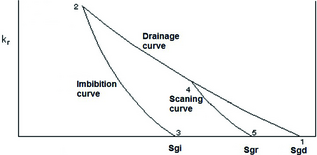Figure 1. Relative permeability model schematic (Carlsons method).

Kleppe et al.  questioned the validity of applying Lands trapping relationship. Kleppes experiments were based on artificial core analysis (Aeroolithe 10), and did not match Lands results. They proposed a correlation based on the maximum residual saturation at the end of complete imbibition :

 $S_{gr}={\frac {S_{gi}}{S_{g}^{max}}}S_{gr}^{max}$
(13)

Carlson  developed a method that produces a scanning curve that is parallel to the imbibition curve. The idea is shifting the imbibition curve horizontally until it intersects the drainage curve (Schlumberger Eclipse technical description) . In this study, Carlsons model has been used to simulate the 2D model. Figure 1 shows how Carlsons method uses drainage, imbibition, and scanning curves to obtain the residual saturation.

### Experimental setup and procedure

#### 2D model setup

The 2D, 31*31 cm model consists of two 3 cm thickness glass plates. A 0.08 cm shim is used for creating a small gap between two surfaces of glass. Using a higher gap between the two glass plates creates difficulties for flow in the model. The model is fitted with a 0.08-cm-diameter plastic fitting that is glued to the internal surface of the shim. The glass plates are glued together by using silicon glue and screws all around the model make it tight. Then, the production and injection ports are installed. To prevent glass bead production, 60-μm nylon filters are installed at the back of the injector and production ports. Glass beads are filled into the model from one corner in a continuous shaking process. The model is vibrated in all the directions in order to make a homogeneous pattern of glass beads.

After filling of the model with glass beads (proxy porous material), the brine proxy fluid is injected into the model. For this purpose, the model is kept vertical and rotated by 45° and the fluid is injected into the model slowly (2 cc/h) from one corner to maintain a uniform filling procedure and avoid air bubble creation. The rotation allowed to fill the model from the lower corner. After filling the model, it is rotated back to its normal position to continue with the experiment.

A pressure transducer with accuracy of 0.01 mbar is used to measure the pressure difference between the model entry and exit. The weight of the injection and production fluids after separation in the separator, are measured and recorded. A digital camera is used for continuous imaging of the tests. Every 30 sec, an image is taken from the test. All the images are used to make a movie of the phenomena but in each drainage and imbibition process six images from different stages are interpreted to obtain the saturation profiles. These images are from the same injection volumes in each test to compare the tests. Each image consists of 6.76 mega pixels. Each pixel is 0.12 mm that is larger than glass beads size (0.07–0.11 mm).

In each test, the CO2 proxy fluid that is dyed in red (by use of Sudan IV (C24H20N4O)) is injected at the bottom of the model in a drainage process to displace the brine proxy fluid. After more than 1.2 pore volume (PV) of injection, the process is changed to imbibition and the brine proxy fluid is injected into the model that is previously saturated with the CO2 proxy fluid. Figure 2 shows the schematic of the model and connections.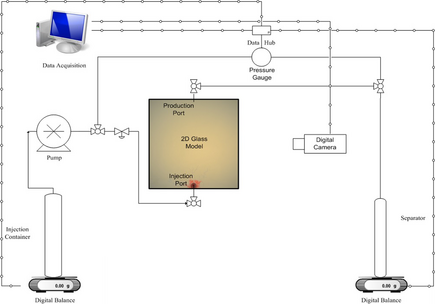Figure 2. Schematic of the 2D glass model.

#### Fluid properties

Two sets of fluids are selected for this 2D experiment at laboratory conditions. The fluid selection is based on scaling analysis. The second fluid set has the same density difference and viscosity ratio but the difference is the wettability behavior to the porous material. The viscosities of the fluid sets are measured by Brookfield LVDV-II+Pro viscometer. Table 1 shows the fluid properties that are measured in atmospheric pressure and 22°C .

Table 1. Fluid properties at laboratory conditions.
Fluid set 1 Fluid set 2
Fluid name n-heptane glycerol (50%) distilled water (50%)a dodecane glycerol (66%) distilled water (34%)a
1. a

Based on weight percent.

Molecular formula C7H16 C3H3O3 + H2O C12H26 C3H3O3 + H2O
Densities (gr/cc) 0.68 1.1 0.78 1.2
Density difference (gr/cc) 0.42 0.42
Viscosities (cp) 0.67 5.8 1.68 14.5
Viscosity ratio ( ${\textstyle {\frac {{\mu }_{Hep.}}{{\mu }_{Gly.+water}}},{\frac {{\mu }_{Dode}.}{{\mu }_{Gly.+water}}}}$ ) 0.1159 0.1155

Ranges of fluid properties for the CO2-brine systems are different for various reservoir conditions but the typical ranges are reported in the literature. Table 2 shows the range of different fluid properties for CO2-brine systems .

Table 2. Fluid properties of CO2 and brine taken from Nordbotten et al.
Cold shallow Cold deep Warm shallow Warm deep
Temperature (°C) 35 85 65 155
Pressure (MPa) 10.5 31.5 10.5 31.5
Density of CO2 (kg/m3) 714 733 266 479
Density of brine (kg/m3) 1121 1099 1104 1045
Viscosity of CO2 (mPa.s) 0.0577 0.0611 0.0395 0.0395
Viscosity of brine (mPa.s) 1.19 0.511 0.687 0.254
Density difference (kg/m3) 407 366 838 566
Viscosity ratio 0.0485 0.1196 0.0575 0.1555

#### Absolute permeability measurements

Absolute permeability measurements are conducted by inserting the same glass beads into a chamber and injecting a brine proxy fluid with different rates and recording the pressure drop .

#### Interfacial tension measurements

Interfacial tension between two sets of fluids has been measured by the pendant drop method. A minimum of one hour is required to allow the drop to be stable in atmospheric pressure and 22°C. Both tests on two fluid sets resulted in the same range of IFT in stabilized period. The IFT estimations from the experiments are in the range of 26 mN/m. Hebach et al.  studied CO2-brine IFT at reservoir pressure and reported values are around 26 mN/m. Chiquet et al. also confirmed the former measurement .

#### Contact angle measurements

An imaging method is used for measuring the contact angle for two sets of fluids. Practically, in porous materials it is difficult to determine the real contact angle because of the pore shapes and complex mineralogy, but in order to obtain an estimation of the range of contact angle and knowledge about the wetting characteristics of each fluid set, the contact angles are measured in the presence of fluid sets and a solid surface of glass . The chamber is filled with the nonwetting phase in each fluid set (n-heptane or dodecane) and a drop of the wetting phase fluid is placed on the glass surface and an enlarged image of the drop is obtained by photography . The experiment continued for minimum one hour to let the droplet stabilize. Very small changes in the angle are observed after the 1 h of stabilization period. A difference of about 16° is observed during two experiments. According to conventional wettability criteria, the wetting phase in fluid set 1 is categorized as strongly wet to the media, while fluid set 2 is considered wet. Figure 3 shows contact angle measurements for two sets of fluids.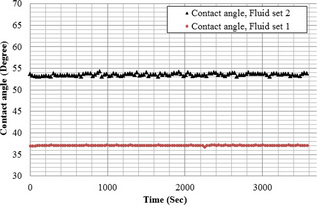Figure 3. Contact angle measurements for two sets of fluids.

## Results

### Absolute permeability results

The absolute permeability calculations are based on injection of the wetting fluid (brine proxy fluid) from fluid set 1 with different injection rates. The pressure drop created by the injection is plotted in Figure 4A. The stabilized pressure in each step gives a point in the pressure versus rate plot, and by use of the Darcy equation absolute permeability is obtained (Fig. 4B). Table 3 shows the absolute permeability measurement conditions.

Table 3. Absolute permeability experimental conditions.
Items Properties
Injection rate (cc/min) 0.1–0.15
System pressure (bar) Atmospheric pressure
Overburden pressure (bar) 10
Experiment temperature (°C) 22
Chamber Length (cm) 5.56
Chamber Diameter (cm) 4.18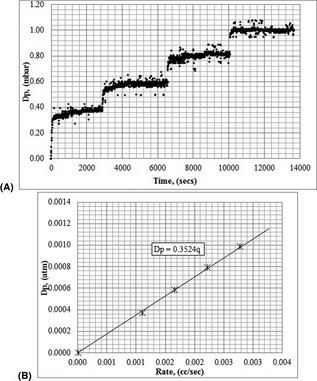Figure 4. (A) Pressure difference by different injection rates (B) stabilized pressure versus injection rate.

The results are in good agreement with the correlation given by Rump and Gupta that is for estimating the absolute permeability in glass bead packs.

 $k={\frac {{\varphi }^{5.5}}{5.6}}d^{2}$
(14)

Here, φ is porosity and d is glass bead diameter in μm and permeability will be estimated in md. Table 3 shows the experimental conditions and Table 4 shows the absolute permeability values from the experiment and correlation.

Table 4. Absolute permeability measurements.
Calculated (k, md) Minimum GB size 70 μm Maximum GB size 110 μm Average
From equation 14 5437 13426 9431
From experiment     7980

### 2D model results

Five successful sets of experiments are carried out in this study. Four tests with fluid set 2 and a test with fluid set 1 are performed. Using two different fluid sets, it is possible to investigate the effect of wettability. The effect of the rate is also studied through performing drainage and imbibition with various rates in test numbers 1 to 4. Table 5 gives a brief description of the tests and calculation of the dimensionless parameters according to the experiment conditions in each test.

Table 5. Successful 2D glass model tests.
Test no. Fluids Drainage rate (cc/min) Imbibition rate (cc/min) Drainage Nc Imbibition Nc Ngr
1 Fluid set 2 0.1 0.1 4.34E-07 3.75E-06 9.74E-01
2 Fluid set 2 0.1 0.15 4.34E-07 5.62E-06 9.74E-01
3 Fluid set 2 0.15 0.1 6.51E-07 3.75E-06 9.74E-01
4 Fluid set 2 0.15 0.15 6.51E-07 5.62E-06 9.74E-01
5 Fluid set 1 0.1 0.1 1.73E-07 1.50E-06 9.74E-01

The experimental design is compared to real CO2 storage sites by use of dimensionless groups in Figure 5. It shows the position of the experiment design and the actual CO2 storage sites. The experimental designs are in good agreement with the real storage conditions. However, attempts to have lower rates to reduce the capillary number failed because of the difficulties for flooding at that condition. Because of the lack of data for contact angles in different CO2 storage projects, dimensionless numbers are calculated without considering the contact angles (Equations 6 and 7). However, in the case of this experiment, dimensionless numbers are calculated both with and without the contact angle in the calculations.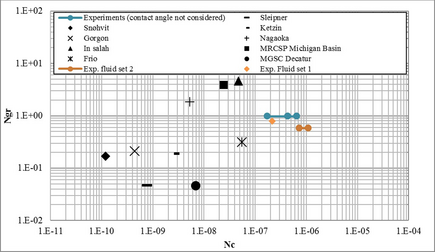Figure 5. Dimensionless groups (Equations 4 and 5) for the experiments and comparison with the dimensionless parameter estimation of some real case CO2 storage projects. Calculations are based on average injection rates and reservoir parameters from published CO2 storage projects [29-32].

#### Results for fluid set 2

Dodecane is injected in the porous material filled with glycerol mixture. In this case, the wettability of the brine proxy fluid is lower than the values in the experiment with fluid set 1. The high viscosity ratio and unfavorable density condition create fingers at the front; however, the overall view of the model shows that the movement of the fluid plume is not dominated by viscous fingers. Close to the injection port the saturation is higher and it is stabilized in the center. CO2 proxy fluid breakthrough is observed before a good sweep of the model and after the breakthrough the pressure gradient will stabilize and minimum change is monitored in the images afterwards. Figure 6 shows some the images that are obtained from the drainage tests in different stages of the injection. Figure 7 shows the pressure drop caused by the injection both in drainage and imbibition.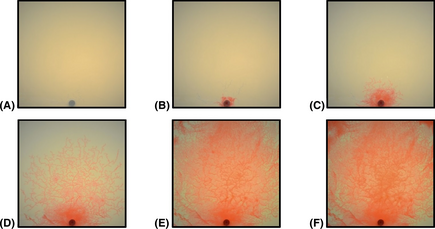Figure 6. Images from drainage process (CO2 proxy fluid is injected), test no. 1 (a) base image (b) 0.007PV injected (c) 0.027 pore volume (PV) injected (d) 0.110PV injected (e) 0.576PV injected (f) 1.201PV injected.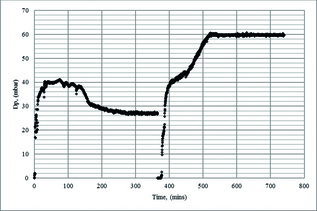Figure 7. Differential pressure in drainage and imbibition processes, test no.1 (discontinuity in the pressure profile is related to changing the process from drainage to imbibition).
Image analysis

To quantify the CO2 plume movement in the drainage and imbibition processes, a green and blue absorption function is defined. A base image before the injection is used to construct the saturation profile. The maximum intensity of green, blue, or red in each pixel is 255, and then the blue and green abortion is as follows :

 ${\mbox{ABS}}=765-({\mbox{Int(blue)}}+{\mbox{Int(green)}})$
(15)

ABS is the absorption of blue and green and Int is intensity in each pixel, and this yields estimations of the saturation if it is scaled within the base image and maximum possible intensity (765). It is also possible to plot the red intensity directly but that technique gives a compact plot of intensity and it is difficult to distinguish the saturations in each time step. Using this method, wide ranges of red intensity are distinguished. To relate the absorption function with CO2 proxy fluid saturation in each pixel, the maximum absorption of blue and green (765) is assigned to maximum saturation and zero saturation is the reading from the base image, then it is possible to find saturation function as below for each pixel:

 $S_{i}={\frac {{\mbox{ABS}}_{i}-{\mbox{ABS}}_{base\quad \quad image}}{765-{\mbox{ABS}}_{base\quad \quad image}}}$
(16)

Si is the CO2 proxy fluid saturation in each pixel and ABSi is the blue and green absorption in each pixel. This method is used for all the pixels from the injection inlet to the production port in the middle of the model, and saturation plots are obtained in Figure 8B from the absorption data in Figure 8A.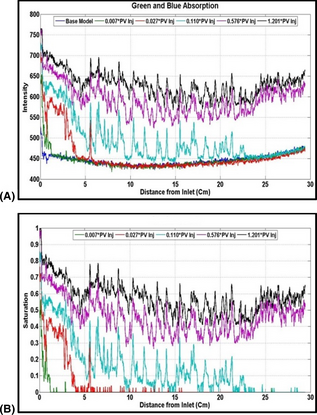Figure 8. Test no. 1, obtained from image processing in drainage process (A) green and blue absorption (B) CO2 proxy fluid saturation.

In the imbibition process the brine proxy fluid front movement is smoother than drainage process as shown in Figure 9. At the tail ending of the plume, trapped portion of CO2 proxy fluid could be distinguished. Injection continued after breakthrough of the brine proxy to let the pressure stabilize and estimations of the trapped volume of the CO2 proxy fluid are obtained. The same image analysis procedure is used to predict the saturations in each pixel. Figure 10A shows the blue and green absorption and Figure 10B shows the saturation profile for the imbibition test in test no 1.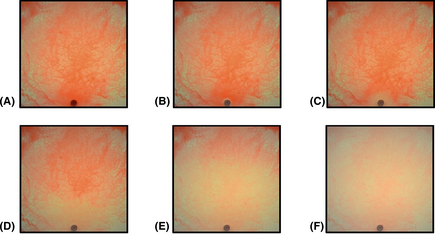Figure 9. Images from imbibition process (brine proxy fluid is injected), test no. 1 (A) base image (B) 0.007 pore volume (PV) injected (C) 0.027PV injected (D) 0.110PV injected (E) 0.509PV injected (F) 1.201PV injected.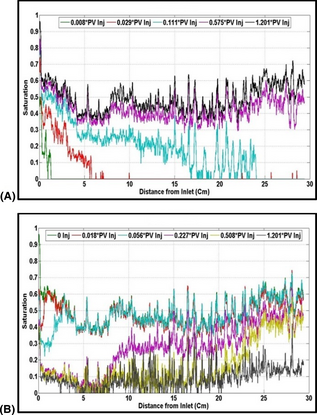Figure 10. Test no. 1, obtained from image processing in imbibition process (A) green and blue absorption (B) CO2 proxy fluid saturation.

#### Rate effect

The effects of the injection rate both for the imbibition and drainage processes are studied through experiments by fluid set 2. In the case of fluid set 1, increasing the rate caused fracturing in the porous material and decreasing the rate caused injection difficulties. So it was easier to control the phenomena for fluid set 2. From the results of image analysis in test numbers 2 and 3, it is observed that higher drainage rates created higher residual saturations, while higher imbibition rates caused lower residual saturations. Test no. 4 verifies these observations, and in the case of higher imbibition and drainage rates, the residual CO2 proxy fluid saturation remains almost in the same range. Although the saturation profiles are not smooth, the average of the data from the image analysis in Table 7 shows the overall behavior in each rate experiment.

#### Results for fluid set 1

The same procedure of flooding and image analysis is performed for the experiment with fluid set 1. The only difference is the wettability of the fluid to the media. Here, the residual values are very sensitive to the fluid changing that is because of the wettability behavior. As it expected, lower residual saturations are observed for the experiments with fluid set 1. In this case, wettability is higher and this helps the nonwetting fluid to flow easier in pore spaces and lower volume is trapped. Figure 11A presents CO2 saturation, obtained from drainage process and Figure 11B is the same function is imbibition process.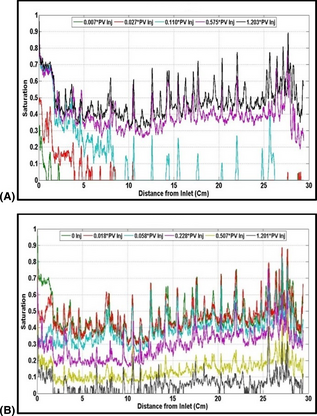Figure 11. Test no. 5 (using fluid set 1) CO2 proxy fluid saturation (A) drainage process, (B) imbibition process.

#### Simulation results

Simulation models are constructed based on the 2D model setup specifications. The Eclipse Black Oil simulator with water–gas fluid system has been used for the simulation studies. In this case, water–gas model is selected but the properties of the gas are set as the CO2 proxy fluid, the reason is that gas–water system is a viable option in the simulator. Table 6 gives the parameters used to construct the model. Relative permeabilities are from the previous measurements in connection with this study  and are presented in Figure 12.

Table 6. Reservoir simulation parameters.
Reservoir parameter Value
Porosity (%) 39.5
Permeability (md) 7890
Temperature (°C) 22
Reservoir pressure (atm) 1
Grid blocks 100 × 1 × 100
DX, DZ (cm) 0.31
DY (cm) 0.08
Model size (cm) 31 × 0.08 × 31
Simulator solver Fully implicit
Fluid system Gas–Water
Units Laboratory
Hysteresis model Carlsons model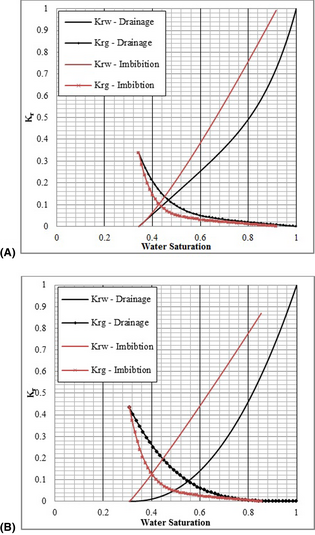Figure 12. Relative permeability (A) fluid set 1, (B) fluid set 2.

The capillary pressure model is obtained based on a modified J function model .

 $P_{C}=\gamma \quad {\mbox{Cos}}\left(\theta \right){\sqrt {\frac {\varphi }{k}}}J\left(s\right)$
(17)
 $J\left(s\right)=A\left(S_{_{\ast }}^{-{\lambda }_{1}}-\right.$$\left.1\right)+B{\left(1-S_{_{\ast }}^{{\lambda }_{2}}\right)}^{1/{\lambda }_{2}}$
(18)

Here A, B, λ1 and λ2 are the fitting parameters, and,

 $S_{_{\ast }}={\frac {S-S_{j}}{1-S_{j}}}$
(19)

Sj is another fitting parameter. According to the Silin experiments , the following parameters are obtained from a drainage experiment on a Frio formation sandstone core, for brine and CO2 at supercritical conditions.

 $A=0.0038;B=0.28;{\lambda }_{1}=3.8\quad and\quad {\lambda }_{2}=$$5.18$

The above correlation with the same fitting parameters is used to construct capillary pressure drainage curves. Imbibition curves for capillary pressure are modeled using the Bentsen and Anli Correlation . Given the threshold pressure Pcd, the generated capillary pressure is as follows:

 $P_{c}=P_{cd}-\lambda log\left(S_{wi}^{_{\ast }}\right)$
(20)
 $S_{wi}^{_{\ast }}={\frac {S_{w}-S_{wi}}{1-S_{wi}}},$
(21)

where λ is capillary pressure normalizing parameter. Figure 13 shows the capillary pressure models for this simulation study, which is constructed, as described above.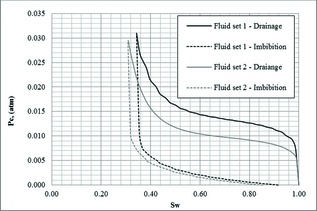Figure 13. Capillary pressure model.

To be able to compare the results of simulation with the images from the laboratory work, the images are converted to so-called solarized pictures by using image processing software. Solarization is a phenomenon in photography in which the image is recorded in a negative and reversed in tone. Then Dark areas appear light or light areas appear dark . In photography this introduces pronounced outlines of highlights, by exposing it briefly to light, then washing and redeveloping, but here in this study, image processing software is used with a constant procedure in order to minimize the effect on the results.

The backgrounds of the images are glass beads filled with the brine proxy fluid that is colorless glycerol and it seems lighter in the images. The back light in the setup makes these sections light, and injected fluid which is dyed in red is darker. Then, in solarized pictures, the injected fluid is red from high saturation to yellow and green for lower saturations and the background is blue. By using this technique, it is possible to compare the results with the simulation in a proper visual demonstration. Figure 14 compares the drainage results for simulation and the pictures. Good agreement is found between overall plume shapes and growth. Figure 15 compares the results for imbibition.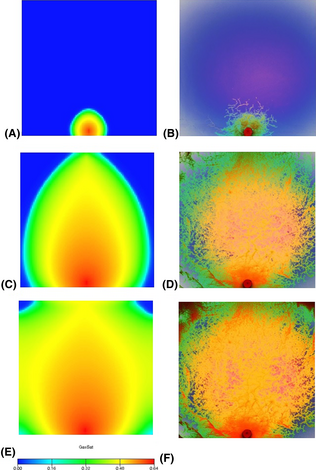Figure 14. Drainage saturation profile from numerical simulation and solarized images from experiment (A) 0.007 pore volume (PV) injected, simulation, (B) 0.007PV injected, image, (C) 0.507PV injected, simulation, (D) 0.507PV image (E) 1.2010PV injected, simulation, (F) 1.2010PV image.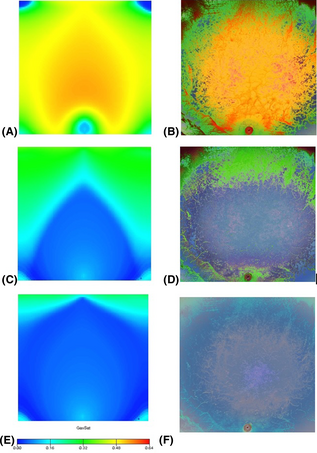Figure 15. Imbibition test saturation profile from simulation and solarized images from experiment (A) 0.007 pore volume (PV) injected, simulation, (B) 0.007PV injected, experiment image, (C) 0.507PV injected, simulation, (D) 0.507PV experiment image, (E) 1.2010PV injected, simulation, (F) 1.2010PV experiment image.

## Discussion

The results from the numerical flow simulations are in good agreement with the results from the image processing method. In general, the overall shape of the CO2 plume is also compatible with the images. However, there is some saturation variations observed in drainage process that is due to capillary fingering. There are also some fine-scale heterogeneities due to the range of glass bead sizes in the model. This might create the tendency for CO2 proxy fluid to flow in easy paths in the porous material. Lenormand et al.  used micromodel to evaluate the microscopic behavior of the liquid flow in the pore spaces. They carried out both drainage and imbibition tests and classified the behavior based on the viscosity ratio and capillary number. They categorized three basic mechanisms, stable front, where the principle force is due to the viscosity of the injected fluid; viscous fingering, where the principle force is due to the viscosity of the displaced fluid; and capillary fingering where at low capillary numbers the principle force is due to the capillary. However, the range of capillary numbers and viscosity ratios are not the same as in this work for CO2 storage, but the overall characteristics are the same. Comparing the results with the Lenormand et al.s shows that the front movement is more under the effects of capillary fingering in both drainage and imbibition process. Figure 16 shows the schematics of the Lenormand et al.s categorization and also the location of the experiments.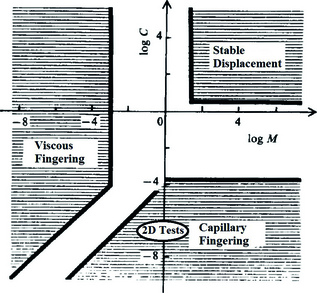Figure 16. Flow regimes phase diagram from Lenormand et al. (1988), ‘2D test’ shows the location of this study.

Figure 17 compares the results from the simulation and image analysis method. In Figure 18, a moving average of the saturations over 100 pixels is used instead of individual pixel calculations. This produces more uniform patterns and it is closer to the results from the Darcy flow models. In fact, numerical simulations also use the concept of averaging in both explicit and implicit methods. In test no. 1 (fluid set 2), less fluctuation is observed and the best match is obtained for the imbibition process in test no. 1.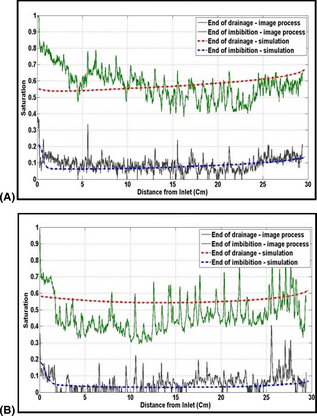Figure 17. CO2 proxy fluid, from image processing method, and numerical simulation, (A) Test no. 1, fluid set 2 (B) Test no. 5, fluid set 1.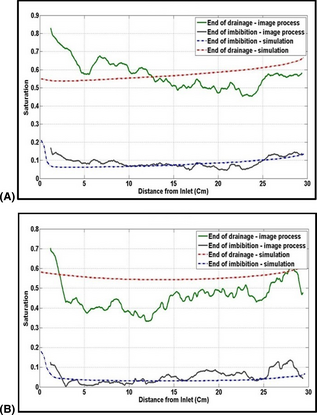Figure 18. CO2 proxy fluid, from image processing method, and numerical simulation, moving average (A) Test no. 1, fluid set 2 (B) Test no. 5, fluid set 1.

At the end of drainage and imbibition processes, Table 7 compares the saturation obtained from the image processing, average saturations obtained from the simulation study and the average of the saturation measurements from image processing in each pixel. Note that the average saturation from simulation and image processing are calculated in the middle of the model (from injection port to producer) but the balance measurements give total saturation in the model.

Table 7. Saturation estimated from the image processing, material balance, and simulation.
Test No Test 1 Test 2 Test 3 Test 4 Test 5
Test mode, d:drainage, i:imbition d i d i d i d i d i
Average value form image analysis 0.578 0.090 0.523 0.081 0.487 0.108 0.493 0.089 0.478 0.053
Average from the simulation 0.575 0.083 0.558 0.040
Reading from the balances (reference measurements) 0.466 0.082 0.469 0.069 0.448 0.093 0.443 0.079 0.442 0.049

### Validity of the results

Analyzing the images from the 2D glass images is very dependent on the picture quality and the analysis technique. High-resolution picturing (camera resolution 16.2 mega pixels) and the RGB color technique in each individual pixel minimize errors. Pressure drop and balance readings from the pressure transducer and volumetric and material balance and visual readings of the production also should be accurate enough to validate the tests. The accuracy of the Fuji electronics pressure drop measurements that is used in this study is in the order of 0.01 mbar, which is acceptable. The production recording is double-checked by both volumetric and visual readings from the separator. These 60-μm nylon filters are used in the chamber at both ends to prevent glass bead production. The filter size has been optimized in order to prevent it from being too fine for the creation of errors in pressure drop and to also to be not too coarse to allow glass bead production. The pressure drop created by this filter is considered to be very small in comparison to the glass bead column pressure drop.

## Conclusions

This study utilizes a 2D model experiment setup to visualize trapping of CO2 proxy fluid in the brine proxy fluid saturated media. The following conclusions are obtained:

1. The use of a 2D glass model, with proxy fluids and porous materials that define the dimensionless groups in the range of real case CO2 storage projects, is an applicable method for better understanding the CO2 injection process and also the residual trapping mechanism.
2. By defining green and blue absorption functions and using the RGB (red, green and blue) color definition technique, the CO2 proxy fluid plume movements in the drainage and imbibition processes is quantified and the residual trapping saturations are estimated.
3. Results of image analyses show that both the imbibition and drainage rates affect the CO2 proxy trapping saturations. It is observed that higher drainage rates created higher residual saturations, while higher imbibition rates caused lower residual saturations.
4. By the use of two different fluid sets with different wettability characteristics in the porous media, the effect of wettability was investigated. By using fluid set 1 with which was more strongly water-wet; considerably lower CO2 proxy residuals are measured. The conclusion was compared with the findings from balance readings and simulation studies.

## Acknowledgments

This publication has been produced with support from the BIGCCS Centre, performed under the Norwegian research program Centres for Environment-friendly Energy Research (FME). The authors acknowledge the following partners for their contributions: ConocoPhillips, Gassco, Shell, Statoil, TOTAL, GDF SUEZ and the Research Council of Norway (193816/S60). Special thanks to Erik Lindeberg, Torleif Holt, Øyvind Haave, Bård Bjørkvik, Szczepan Polak and Svein Arild Utne for invaluable technical supports, help and advice in SINTEF petroleum research.

## Conflict of Interest

None declared.

### Document informationPublished on 01/06/17
Submitted on 01/06/17

Licence: Other

### Document Score0

Views 11
Recommendations 0